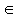# Bolzano Theorem (BT)

Let, for two real a and b, a < b, a function f be continuous on a closed interval [a, b] such that f(a) and f(b) are of opposite signs. Then there exists a number x0[a, b] with f(x0)=0.## Intermediate Value Theorem (IVT)

Let, for two real a and b, a < b, a function f be continuous on a closed interval [a, b] such that f(a)<f(b). Then for every y0 such that f(a)<y0<f(b) there exists a number x0[a, b] with f(x0)=y0.Clearly BT is only a special case of IVT. However it's interesting that the more general IVT could be deduced from its special case, BT. Indeed, let f(a)<y0<f(b). Introduce a new function g(x)=f(x)-y0. Then it follows that g(a)=f(a)-y0<0 whereas g(b)=f(b)-y0>0. Therefore, g(a) and g(b) are of opposite signs. Additionally, g is continuous wherever f is. In particular, g is continuous on [a, b] and thus satisfies the conditions of BT. Therefore, there exists x0[a, b] such that g(x0)=0. Written explicitly this says f(x0)-y0=0 or, finally,

f(x0)=y0

Q.E.D.

A complete proof of the Bolzano Theorem appeares elsewhere.### Related materialRead more...

• Cavalieri's Principle
• Derivative of Sine and Cosine
• Distance From a Point to a Straight Line
• Estimating Circumference of a Circle
• Maximum Volume of a Cut Off Box
• Mistrust Intuition of the Infinite
• Naturally Discontinuous Functions
• Rolle's and The Mean Value Theorems
• Function, Derivative and Integral
• Area of a Circle by Rabbi Abraham bar Hiyya Hanasi
• Schwarz Lantern
• Two Circles and a Limit
• Deceptive Appearances
• Problem 4010 from Crux Mathematicorum
•